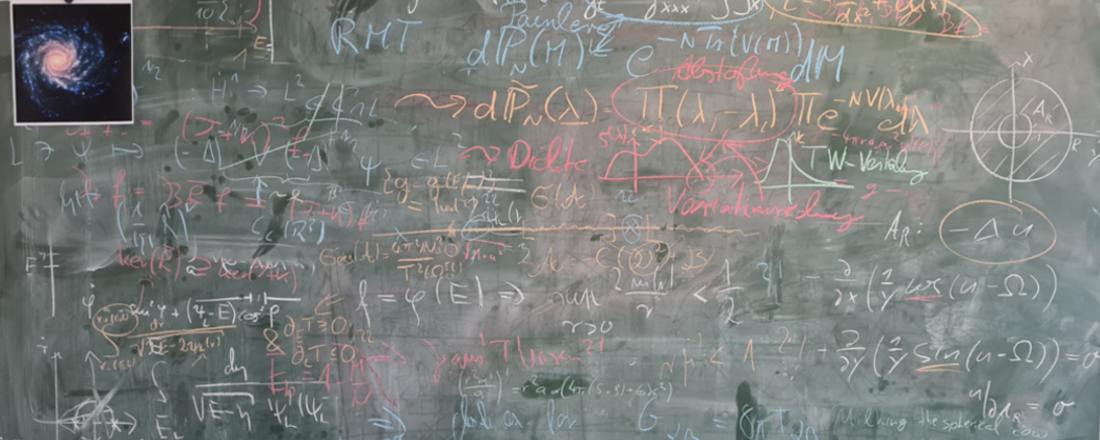## Faculty of Mathematics, Physics and Computer Sciences

### Chair of Mathematics VI Nonlinear Analysis and Mathematical Physics

Print page## ​Welcome to the Chair of Nonlinear Analysis and Mathematical Physics

The chair works on mathematical problems motivated by physics. In particular, kinetic models from general relativity and stellar dynamics, stability problems in hydrodynamics and magnetohydrodynamics, nonlinear and stochastic phenomena of wave propagation using integrable systems, and universal properties of local statistics of random matrices are studied. Primarily methods of nonlinear analysis but also numerical simulations are used.

Webmaster: Univ.Prof.Dr. Thomas Kriecherbauer